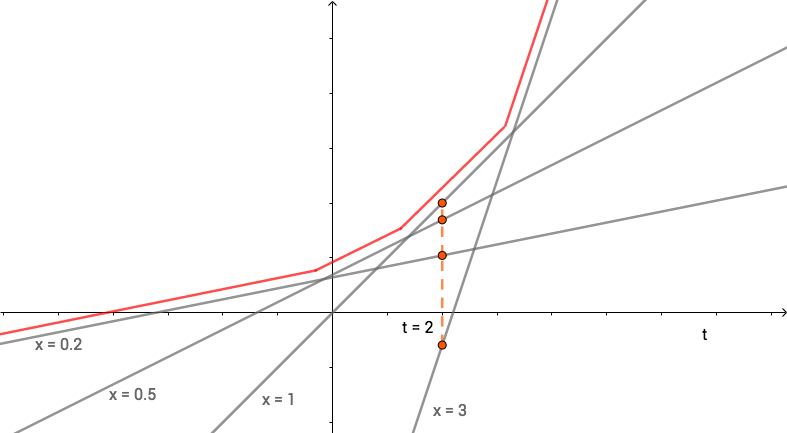f-divergence GAN 是对 GAN 框架的理论统一，本文学习过程中的一些笔记，包括基本公式的推导和重要概念的理解。

## GAN 的基本思想(图片来源：https://blog.openai.com/generative-models/)## GAN 的算法

• 初始化 D, G 的参数 $\theta_d$ 和 $\theta_g$
• 每一个迭代中：
• 从真实数据的分布 $P_{data}(x)$ 中采样 $m$ 个样本 $\{x^1, x^2, \dots, x^m\}$
• 从先验的噪声分布 $P_{prior}(z)$ 中采样 $m$ 个样本 $\{z^1, z^2, \dots, z^m\}$
• 将噪声输入生成器 G，生成样本 $\{\tilde{x}^1, \tilde{x}^2, \dots, \tilde{x}^m\}, \tilde{x}^i = G(z^i)$
• 更新判别器 D 的参数，即最大化：
• $\tilde{V} = \frac{1}{m}\sum_{i=1}^m{\log D(x^i)} + \frac{1}{m}\sum_{i=1}^m{\log (1-D(\tilde{x}^i))}$
• $\theta_d\leftarrow\theta_d+\eta\nabla\tilde{V}(\theta_d)$
• 从先验的噪声分布 $P_{prior}(z)$ 中 采样 $m$ 个样本 $\{z^1, z^2, \dots, z^m\}$
• 更新生成器 D 的参数，即最小化：
• $\require{cancel}\tilde{V} = \cancel{\frac{1}{m}\sum_{i=1}^m\log D(x^i)} + \frac{1}{m}\sum_{i=1}^m\log (1-D(G(z^i)))$
• $\theta_d\leftarrow\theta_d-\eta\nabla\tilde{V}(\theta_d)$

## ƒ-divergence

In probability theory, an ƒ-divergence is a function $D_f(P||Q)$ that measures the difference between two probability distributions $P$ and $Q$. It helps the intuition to think of the divergence as an average, weighted by the function f, of the odds ratio given by $P$ and $Q$.

$$D_f(P||Q)=\int_xq(x)f(\frac{p(x)}{q(x)})dx$$

### 为什么 $D_f$ 可以衡量距离？

$$D_f(P||Q)=\int_xq(x)\underbrace{f(\overbrace{\frac{p(x)}{q(x)}}^{=1})}_{=0}dx=0$$

$$\require{cancel} \begin{eqnarray} D_f(P||Q) &=& \int_xq(x)f(\frac{p(x)}{q(x)})dx \\ &\ge& f(\int_x\cancel{q(x)}\frac{p(x)}{\cancel{q(x)}}dx)=f(1)=0 \end{eqnarray}$$

### 一些 ƒ-divergence

$$D_f(P||Q)=\int_x q(x)\frac{p(x)}{q(x)}\log(\frac{p(x)}{q(x)})dx=\int_xp(x)\log(\frac{p(x)}{q(x)})dx$$

$$D_f(P||Q)=\int_xq(x)(-\log(\frac{p(x)}{q(x)}))dx=\int_xq(x)\log(\frac{q(x)}{p(x)})dx$$

$$D_f(P||Q)=\int_x q(x)(\frac{p(x)}{q(x)}-1)^2dx = \int_x\frac{(p(x)-q(x))^2}{q(x)}dx$$

### ƒ-divergence 不是距离

1. $d(x, y) \ge 0$ 非负性
2. $d(x, y) = 0$ 当且仅当 $x = y$
3. $d(x, y) = d(y, x)$ 对称性
4. $d(x, z) \le d(x, y) + d (y, z)$ 三角不等式

Jensen–Shannon (JS) Divergence就满足所有条件。一如既往，想看证明，请查看 原论文

## Fenchel Conjugate

Conjugate 翻译是“共轭”，不明觉厉。对于每个凸函数，我们都可以 定义 一个它的共轭函数：

$$f^*(t) = \max_{x\in \mathbf{dom}(f)}\{xt-f(x)\}$$$$f^*(t) = \max_{x\in \mathbf{dom}(f)}\{xt-f(x)\} \Longleftrightarrow f(x) = \max_{t\in \mathbf{dom}(f^*)} \{xt-f^*(x)\}$$

## ƒ-divergence 与 GAN

\begin{eqnarray} D_f(P||Q) &=& \int_xq(x)f(\frac{p(x)}{q(x)})dx \\ &=& \int_xq(x)\left(\max_{t\in\mathbf{dom}(x^* )} \left\{\frac{p(x)}{q(x)}t-f^*(t)\right\}\right)dx \end{eqnarray}

\begin{eqnarray} D_f(P||Q) &\ge& \int_xq(x)\left(\frac{p(x)}{q(x)}D(x)-f^*(D(x))\right)dx \\ &=& \underbrace{\int_xq(x)D(x)dx - \int_xq(x)f^*(D(x))dx}_{M} \end{eqnarray}

\begin{eqnarray} D_f(P||Q) &\approx& \max_D\int_xp(x)D(x)dx-\int_xq(x)f^*(D(x))dx \\ &=& \max_D\left\{\underbrace{E_{x\sim P}[D(x)]}_{\text{Samples from P}} - \underbrace{E_{x\sim Q}[f^*(D(x))]}_{\text{Samples from Q}} \right\} \end{eqnarray}

\begin{align} G^*&=\arg\min_GD_f(P_{data}||P_G) \\ &=\arg\min_G\max_D{E_{x\sim P_{data}}[D(x)]-E_{x\sim P_G}[f^*(D(x))]} \\ &= \arg\min_G\max_DV(G, D) \end{align}

## 小结

ƒ-divergence GAN 是对 GAN 模型的统一，对任意满足条件的 $f$ 都可以构造一个对应的 GAN。

GAN 的目的是训练生成器 D 使之生成的数据对应的分布 $P_G$ 与真实数据的分布 $P_{data}$ 尽可能接近，即最小化 $D_f(P||Q)$。然而我们无法确切算出 $p(x)$ 及 $q(x)$，因此我们通过 Conjugate 将求 $D_f(P||Q)$ 转变成一个优化问题，于是我们的目标变成找到一个合适的函数 $D$ 来逼近 $D_f(P||Q)$。